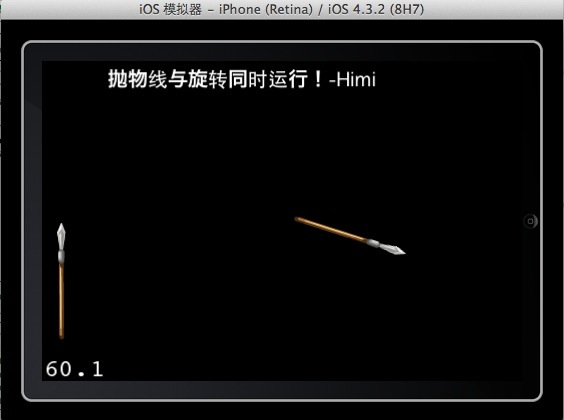## 【iOS-Cocos2d游戏开发之十三】CCSprite利用Bezier（贝塞尔）做抛物线动作并让CCSprite同时播放两个Action动作！

junwong 发布于 2012/03/02 16:15

 1 2 3 4 5 6 7 8 9 10 11 12 13 14 15 16 17 18 `//  抛物线    -Himi` `//mSprite：需要做抛物线的精灵` `//startPoint:起始位置` `//endPoint:中止位置` `//dirTime:起始位置到中止位置的所需时间` `- (` `void` `) moveWithParabola:(CCSprite*)mSprite startP:(CGPoint)startPoint endP:(CGPoint)endPoint dirTime:(` `float` `)` `time` `{` `    ` `float` `sx = startPoint.x;` `    ` `float` `sy = startPoint.y;` `    ` `float` `ex =endPoint.x+50;` `    ` `float` `ey =endPoint.y+150;` `    ` `int` `h = [mSprite contentSize].height*0.5;` `    ` `ccBezierConfig bezier; ` `// 创建贝塞尔曲线` `    ` `bezier.controlPoint_1 = ccp(sx, sy); ` `// 起始点` `    ` `bezier.controlPoint_2 = ccp(sx+(ex-sx)*0.5, sy+(ey-sy)*0.5+200); ` `//控制点` `    ` `bezier.endPosition = ccp(endPoint.x-30, endPoint.y+h); ` `// 结束位置` `    ` `CCBezierTo *actionMove = [CCBezierTo actionWithDuration:` `time` `bezier:bezier];` `    ` `[mSprite runAction:actionMove];` `}`

//—-  OK，下面来介绍第二个知识点：

OK，看下封装角度后的抛物线并同时旋转方法如下:

 1 2 3 4 5 6 7 8 9 10 11 12 13 14 15 16 17 18 19 20 21 22 23 24 25 26 `//  抛物线运动并同时旋转    -Himi` `//mSprite：需要做抛物线的精灵` `//startPoint:起始位置` `//endPoint:中止位置` `//startA:起始角度` `//endA:中止角度` `//dirTime:起始位置到中止位置的所需时间` `- (` `void` `) moveWithParabola:(CCSprite*)mSprite startP:(CGPoint)startPoint endP:(CGPoint)endPoint startA:(` `float` `)startAngle endA:(` `float` `)endAngle dirTime:(` `float` `)` `time` `{` `    ` `float` `sx = startPoint.x;` `    ` `float` `sy = startPoint.y;` `    ` `float` `ex =endPoint.x+50;` `    ` `float` `ey =endPoint.y+150;` `    ` `int` `h = [mSprite contentSize].height*0.5;` `    ` `//设置精灵的起始角度` `    ` `sprite.rotation=startAngle;` `    ` `ccBezierConfig bezier; ` `// 创建贝塞尔曲线` `    ` `bezier.controlPoint_1 = ccp(sx, sy); ` `// 起始点` `    ` `bezier.controlPoint_2 = ccp(sx+(ex-sx)*0.5, sy+(ey-sy)*0.5+200); ` `//控制点` `    ` `bezier.endPosition = ccp(endPoint.x-30, endPoint.y+h); ` `// 结束位置` `    ` `CCBezierTo *actionMove = [CCBezierTo actionWithDuration:` `time` `bezier:bezier];` `    ` `//创建精灵旋转的动作` `    ` `CCRotateTo *actionRotate =[CCRotateTo actionWithDuration:` `time` `angle:endAngle];` `    ` `//将两个动作封装成一个同时播放进行的动作` `    ` `CCAction * action = [CCSpawn actions:actionMove, actionRotate, nil];` `    ` `[mSprite runAction:action];` `}`